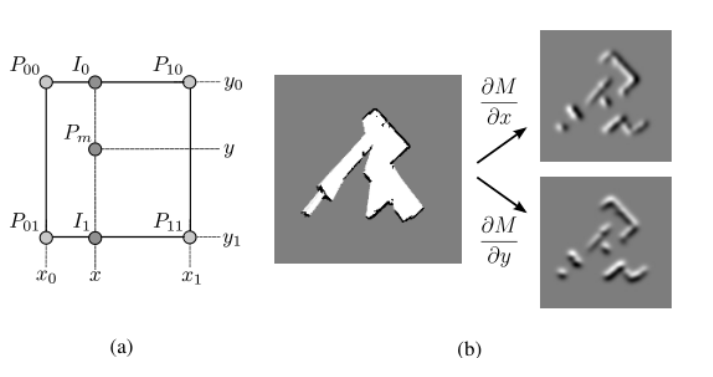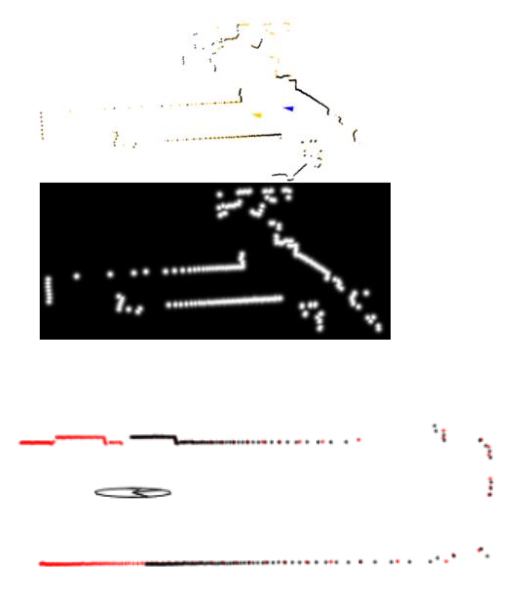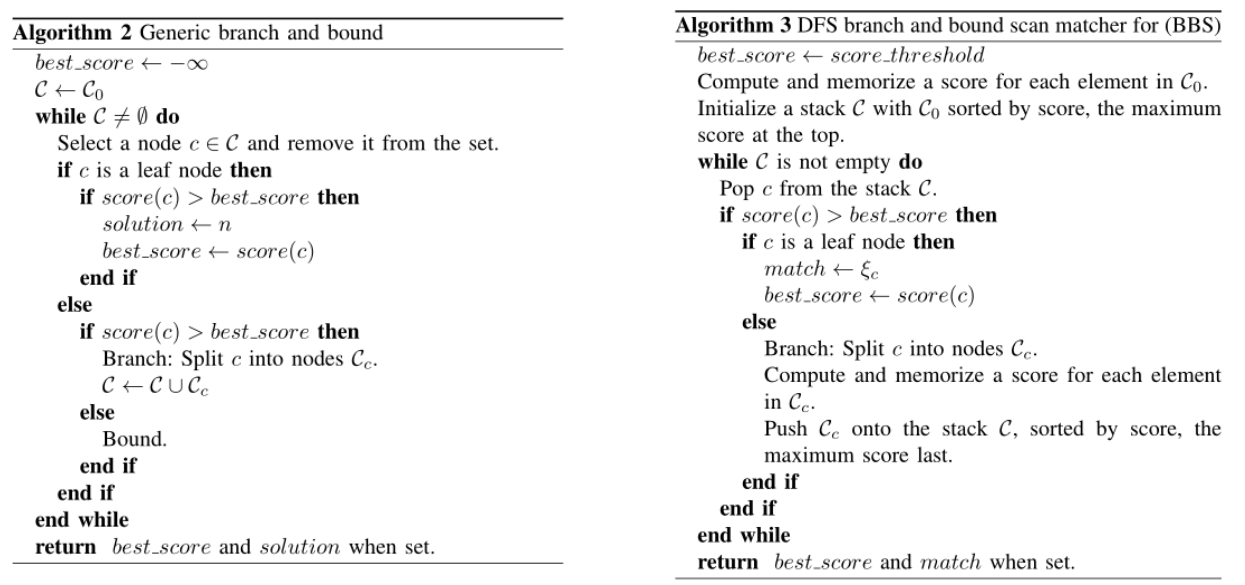0%

## 高斯牛顿优化方法

### 基本原则 & 数学描述

HectorSLAN 使用的是这种方法。根据基于优化方法，只要给定一个目标函数，我们可以把激光帧间的匹配问题转换为求解目标函数的极值问题（通常是求最小值）：

### 地图双线性插值## NDT (Normal Distribution Transform) 方法

### 基本思想

NDT 方法的基本思想是将空间划分成一个个 cell，这里的 cell 的概念要和栅格 grid 区分一下，通常单个栅格会取很小，其只代表了一个值。相对而言，cell 更像是一个小区域。有了各个 cell 之后为每一个 cell 定义一个高斯分布用来替代势场，因此整个空间里面就形成了一个分段连续的势场（在各自的 cell 里面连续）。通过这样的方法，结合连续的势场我们可以直接用牛顿法进行迭代（Hessian 矩阵非常好求），同时这样一个势场也不受离散化的影响。

### 数学描述

$T = (tx, t_y, t\theta)$ 表示需要求解的值

$x_i = (x, y)^T$ 表示激光点坐标

\begin{aligned} T(x_i) = \begin{bmatrix} \cos{\theta} & -\sin{\theta} \ \sin{\theta} & \cos{\theta} \end{bmatrix} \begin{bmatrix} x_i \ y_i \end{bmatrix} + \begin{bmatrix} t_x \ t_y \end{bmatrix} \end{aligned}

$q_i$, $\Sigma_i$ 分别表示 $x_i$ 对应点的高斯分布的均值和方差，则当前激光点的的得分为(得分越小，匹配程度越高)：

### 算法流程

• 对环境进行 cell 的划分，为每一个 cell 构造一个高斯分布
• 根据初始解把当前帧的激光点转换到参考帧上，并确定属于哪个 cell
• 用牛顿法进行迭代求解

## 相关匹配方法及分枝定界加速 （CSM）

### 相关方法——算法流程• 如上图所示，首先我们需要对地图通过高斯模糊来构造似然场
• 在一个指定的搜索空间（比如 1m×1m，30°范围内）来进行搜索，这里可以有不同搜索的方法，后面会详细描述；对搜索得到的每一个位姿进行得分计算（匹配程度）
• 根据上一步得到位姿得分，计算本次位姿匹配的方差

### 相关方法——位姿搜索

1. 暴力搜索：通过多层循环（对 $(x, y, \theta)$ 而言是三层）枚举每一个位姿,分别计算每一个位姿的得分,计算量巨大。因为激光雷达数据在每一个位姿都要重新投影,投影需要计算 sin 和 cos 函数（循环顺序是： $x\rightarrow y\rightarrow \theta$）;
2. 预先投影搜索：把暴力搜索中的三层循环 $(x, y, \theta)$ 交换一下顺序,最外层对 $\theta$ 进行搜索,这样内层 $x, y$ 的投影不需要单独计算 sin 和 cos 值，需要计算 sin 和 cos 函数的位姿数从 $nxn_yn\theta$ 降为 $n_\theta$ 。能极大的加速算法的运行速度;
3. 多分辨率搜索：
• 构造粗分辨率 25cm 和细分辨率 2.5cm 两个似然场
• 首先在粗分辨率似然场上进行搜索,获取最优位姿
• 把粗分辨率最优位姿对应的栅格进行细分辨率划分,然后再进行细分辨率搜索,再次得到最优位姿。
• 要求：粗分辨率地图的栅格的似然值为对应的细分辨率地图对应空间的所有栅格的上界

### 分枝定界算法——基本思想

• 分枝：跟节点表示整个解空间，深度为 1 的节点表示解空间的 n 个子空间之一（分辨率是根节点的 1 / n），以此类推。将解空间层层划分直至到真实解（分辨率达到所需要求）即叶节点。
• 定界：要实现通过剪枝来进行加速（dfs 搜索），对于某个子树而言，必须保证该子树根节点的值是该子树所有节点的值的上界（如果是求解最小值问题就是下界）；这样在搜索过程过程中只需要维护一个当前最优值，当遇到某个节点的值低于该值，我们就不需要对以该节点为根节点的子树进行搜索，从而进行加速。

### 分枝定界算法——算法流程